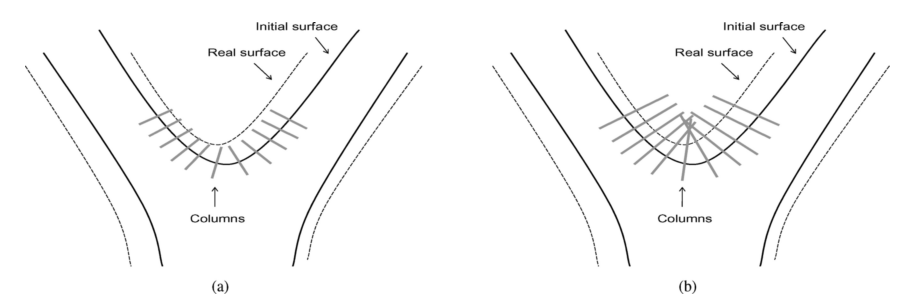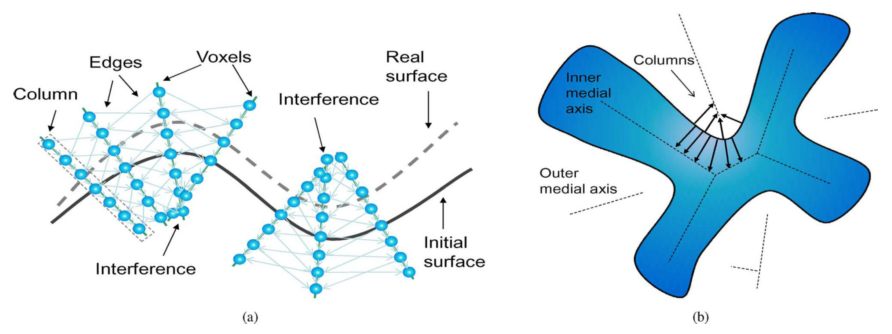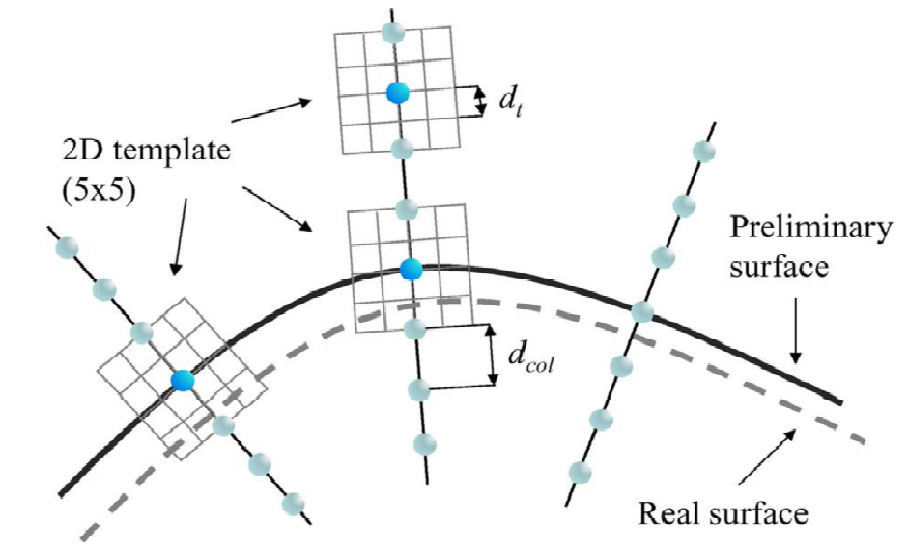Jarvis' Blog 总有美丽的风景让人流连

# 基于图搜索的三维医学图像分割方法 (二)

2017-10-20
Jarvis
Post

## 基于图搜索的分割

• 原始图像的预分割及其曲面的网格表示,
• 图像的重采样,
• 图的构建,
• 图的搜索.

### 基于中轴的图像重采样• 体素列的长度太短, 以至于图无法抓取到曲面足够的信息;
• 体素列的长度太长, 以至于与其他的体素列相交, 如图1(b)所示. 显然气管分叉的角度太小和较为末端的支气管处必然会发生这种情况.

• 使用预分割表面的中轴线来帮助避免体素列的相交;
• 对预分割表面做膨胀操作, 保证不干扰的体素列足够长从而能够包含最优位置, 同时消除尖角的情况.

• 记预分割表面的网格点的集合为 $S$ , 然后计算 $S$ 的泰森多边形(Voronoi diagram, VD)和对偶的德劳内三角剖分(Delaunay triangulation)D;
• 对每个顶点均求一对 $v_{p_1}$ 和 $v_{p_2}$ 分别作为内中轴线和外中轴线, 这两点选为最大的德劳内球的球心(德劳内四面体的外切球球心);
• 选择球心是选择点 $p$ 的 $k$ 个最近邻中最大的值;
• 体素列的长度由体素到中轴线的距离决定.### 损失函数

$\text{Cost}_{\text{outer}}(v) = \omega\cdot I_t(v)*M_{Sobel_1} = (1-\omega)\cdot I_t(v)*M_{Marr},$ $\text{Cost}_{\text{inner}}(v) = \omega\cdot I_t(v)*M_{Sobel_2} = (1-\omega)\cdot I_t(v)*M_{Marr}.$Content